# ISEE Middle Level Math : How to find median

## Example Questions

← Previous 1 3 4 5 6 7 8 9 10 11

### Example Question #1 : Median

On the last day of school, Adam asked each of his classmates how many days of school they missed for the entire school year.  He created a stem-and-leaf plot with the information.

0 | 0 0 2 4 5 6 8

1 | 0 0 1 2

2 | 3

4 | 7

What is the median number of school days missed by Adam's classmates?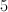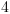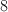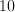Explanation:

In a stem-and-leaf plot, each number on the right side of the vertical lines is a piece of data.  For instance, the "4 | 7" means one student missed 47 days(!) of school.  Each number on the left of the line represents the ten's digit, and each number on the right of the line represents the one's digit.

We can find the total number of classmates by counting the numbers on the right side of the vertical lines, which in this case is 13.

The median of a group of data represents the physical middle of the data.

If there is an even number of pieces of data, you take the middle two pieces of data, add them up, and divide by two for the median.

If there is an odd number of pieces of data (such as here), one number represents the median.  Since we have 13 pieces of data, the 7th value is the median, because we would have 6 pieces smaller or equal to it, and 6 pieces greater than or equal to it.

The seventh piece of data here is the 8, which is "0 | 8".  Since the ten's digit is 0, that means that the median number of days missed is 8.

### Example Question #1 : Median

Find the median of this set of numbers: 89, 75, 111, 94, 36, 89, 81.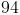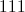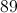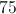Explanation:

Place the numbers in order from least to greatest:

36, 75, 81, 89, 89, 94, 111

Identify the middle number in the set. The answer is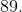### Example Question #3 : How To Find Median

Find the median in this set of numbers:

3021, 3211, 3120, 3102, 3012, 3201, 3210, 3112, 3011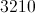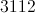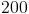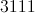Explanation:

First, order the numbers from least to greatest: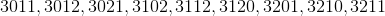Then, identify the middle number: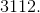### Example Question #2 : Median

Subtract the median from the mode in this set of numbers:

241, 244, 422, 424, 422, 214, 412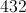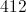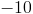Explanation:

First, order the numbers from least to greatest: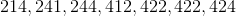Identify the mode (most frequently appearing number):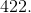Identify the median (middle number):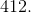Finally, subtract the median from the mode: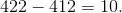### Example Question #1 : Median

Find the median in this set of numbers:

1144, 1187, 1198, 1034, 1073, 1187, 1081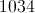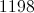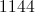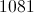Explanation:

First, order the numbers from least to greatest.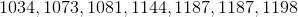Identify the middle number in the set.

### Example Question #6 : How To Find Median

Find the median in this set of numbers:

2521, 2512, 2525, 2552, 2551, 2525, 2515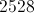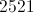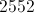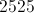Explanation:

First, order the numbers from least to greatest: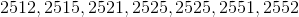Identify the middle number: 2525

### Example Question #2 : Median

Find the median in this set of numbers:

9881, 9889, 9818, 9981, 9891, 9918, 9989, 9889, 9198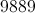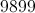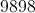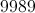Explanation:

First, order the numbers from least to greatest: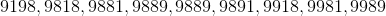Identify the middle number: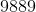### Example Question #2 : Median

Find the median in this set of numbers:

3343, 4434, 4334, 3343, 4343, 3434, 3334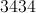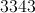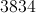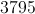Explanation:

First order the numbers from least to greatest: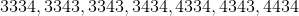Then, identify the middle number: 3434.

### Example Question #1 : Median

Find the median in this set of numbers:

6363, 6336, 6366, 6633, 6333, 6363, 6663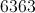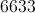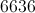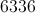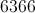Explanation:

First, order the numbers from least to greatest: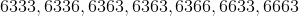Then, identify the middle number: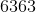Answer: the median is### Example Question #10 : How To Find Median

Find the median in this set of numbers:

9080, 9008, 9800, 9099, 9009, 9090, 9008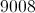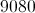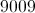Explanation:

First, order the numbers from least to greatest: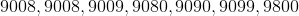Then, identify the middle number: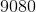.